﻿ Superimposed Decomposition of Wavelet Analysis for Seismological Investigations: Validation on GPS Stations Displacements in Central Alaska (2008-2012)Publications are Open
Access in this journal
Article Versions
Export Article
• Normal Style
• MLA Style
• APA Style
• Chicago Style
Special Issue
Open Access Peer-reviewed

### Superimposed Decomposition of Wavelet Analysis for Seismological Investigations: Validation on GPS Stations Displacements in Central Alaska (2008-2012)

Journal of Geosciences and Geomatics. 2017, 5(3), 147-166. DOI: 10.12691/jgg-5-3-6
Published online: July 17, 2017

### Abstract

The comprehensive study of seismic waves is very important in order to understand the complex dynamic processes of the Earth’s interior as well as its signals emerged to the physical surface. In the last three decades, observational Global Positing System (GPS) products through determining the displacements of ground GPS station in horizontal and vertical directions have widely been applied to infer the tectonic stress regimes generated by the subsurface processes ranging from the local fault systems to the huge tectonic plate movements. However, the complex patterns generated during such movements are not always easy to interpret. Therefore, it is necessary to develop new approaches by modifying the previous strategies and improve the current methodologies to understand better such sudden crustal movements. In this paper, we employed 5 years GPS stations displacements data from January 1, 2008 to December 31, 2012 in the seismically active central Alaska area, in order to get the average daily and annual velocities of the GPS stations. Then, vector summation for horizontal and vertical velocities has been applied to yield the total velocities of GPS stations displacements. Moreover, we applied the Cross-Correlation Functions (CCFs) analysis to recognize the significant and homogenous displacements among the total displacements of GPS stations located in this region to be employed in next step for the superimposed decomposition of wavelet analysis at level number 1 and 2. Finally, the normal probability histograms related to the accuracy of each analysis are calculated and presented in details. The results show a very good agreement between the CCFs reorganizations, proposed wavelet decomposition methodology, and simultaneous earthquakes regimes occurred in central Alaska from 2008 to 2012 year.

### 1. Introduction

Alaska is a unique and complex tectonic area 1 since its southern edge is located on the boundary between the North American Plate and the Pacific Plate. Relative to the North American Plate, the Pacific Plate is moving northwestward in subduction at the annual average rate of 5-7 centimeters 5. In fact, the area of Alaska is formed by several active faults, like Fair-weather fault, Denali fault, Castle mountain fault, Tintina fault and Totschunda fault. Thus, the stress released by these faults is responsible, in about twenty centuries, for the generation of many big earthquakes 18, 28, 29. These essential faults are but not limited to Denali fault where several events with a magnitude higher than 6 occurred over the last century as Mw=7.2 in July 7, 1912, Mw=6.2 in August 31, 1958, Mw=6.7 in October 23, 2002 and Mw=7.9 in November 3, 2002 43.

Although a complete set of information about Alaska tectonic regime has been achieved from 1992 to 2007 by using GPS data 18, most of recent studies have focused on the southern part of Alaska where the spatial distribution of GPS sites is denser than the other parts. In particular, it has been found that the velocity pattern of the GPS stations located across south and central Alaska is spatially complex 9, 19. This is due to the influence of several different significant contributions to the crust deformation 2, 23.

Consequently, monitoring the seismicity requires more robust and sensitive methods in general 27, 32. In this study, it is initially assumed that the pre-seismic, co-seismic and post-seismic shocks related to the Earth’s crustal deformations have been considered as a single earthquake event. Cross-correlation functions (CCFs) into GPS stations displacements are applied by using the data from five GPS stations located at central Alaska (AC15, AC20, ATW2, CLGO and SG27). The daily GPS stations displacements are limited to the period ranging from January 1, 2008 to January 1, 2013. These geodetic GPS stations are equipped with continuous GPS receivers which can collect data in each 15-second 21, 22, 31.

Firstly, we analyze the daily horizontal (both North-South and East-West) and vertical (upwards or downwards) GPS vector displacements for the group of events with Mw≥4 and their corresponding depth for the period ranging from January 1 2008 to December 31 2012. Cross-correlation values between the GPS station displacements are then computed to retrieve those significant and homogenous movements among the selected GPS stations. Moreover, the annual horizontal and vertical velocities are computed and presented for each year in details. Finally, by applying the superimposed decomposition of wavelet analysis on vertical normalized cross-correlation values, the fit between the occurred events and their depth as well as the designed retrieval wavelet 15, 38 were analyzed. The accuracy of these results, especially in level number 1 and level number 2 were discussed mainly through probability histograms of convergent decomposition 32, 33, 35.

Practically, the wavelet transforms (both discrete and continuous) can localize information in time as well as frequency space 16, 37, simultaneously; and therefore providing a powerful technique for analyzing the seismic data 17, 36. Furthermore, using the CCFs data on the GPS stations can yield us with correlated values where the homogenous region of central Alaska has very similar tectonic characteristic with a good approximation, seismically. Conversely, the existing methods and approaches (mostly those numerical analyzing mythologies except spectral ones such as interferometric) usually focus on station by station’s movements which may need a huge amount of computations. In addition to this, the interferometric approaches are prodigal as well as holding low resolutions in both spatial and temporal scale.

According to the above discussion, Section 2 describes theory and methodology; used data and observations are presented in Section 3; Section 4 includes results and discussions. Finally, summary and conclusions are discussed in Section 5.

### 2. Theory and Methodology

The flowchart of the proposed methodology is illustrated in Figure 2. As shown in the Figure 2, the suggested approach followed in this study includes the following steps: processing GPS raw data derived from continuous GPS stations located in central Alaska; computing horizontal and vertical displacements based on ephemeris and baseline measurements; comparing the GPS stations displacements with the recent earthquakes occurred in Alaska by considering a main group of events with Mw ≥4 and their depth values; extracting total horizontal and vertical velocities as daily and yearly average values; applying cross-correlation functions (CCFs) between the GPS stations displacements to get the coefficients of homogenous irregularity on the selected stations for each year in three directions; developing wavelet analysis based on normalized vertical displacements using superimposed decomposition in two levels and eventually displaying annually horizontal and vertical movements for each station during 2008, 2009, 2010, 2011 and 2012 year.

• Figure 1. Locations of GPS ground stations including AC15, AC20, ATW2, CLGO and SG27 at central Alaska, the United States of the America
• Figure 2. Flowchart of proposed methodology for analyzing GPS stations displacements and recent earthquakes reports in central Alaska

In the following sections, the velocity computations, CCFs of stations displacements and wavelet analysis are being expatiated.

2.1. Average Horizontal, Vertical and Total Velocity

In order to compute horizontal, vertical and total daily and annual velocity of each GPS station’s movements, the total regression solution is proposed as the following: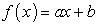(1)

where f(x) stands for north-south, east-west or vertical displacement of the GPS station (mm), x is time (day), a is the velocity of the station (mm/day) and b is the intercept parameter, which has no practical meaning here. It is initially assumed that the displacements of GPS stations satisfy the normal function distribution 14, 20. The right side of equation (1) is computable by applying the total linear regression on the entire daily displacements of one GPS station during a year. As it can be understood from equation (1), the GPS station’s movements towards north, east or upward, takes positive values, while the one towards south, west or downward, would take negative values. It is clear that the average annual velocities can easily be computed then by multiplying the average daily velocities by 365.25.

The orientation of the daily GPS velocity vector can be computed by applying equation (2) to the total displacements of the GPS station as: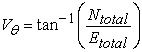(2)

where Vθ stands for the angle of GPS velocity vector starting from east direction counterclockwise, Ntotal and Etotal are the average annual velocities of GPS station in the north-south and east-west directions, respectively (mm/year). Then, the length of the GPS station’s velocity vector would be the quadratic sum of these elements.

2.2. Cross-correlation Functions (CCFs) of GPS Station’s Displacements

If we consider a multivariate time series Vt with the corresponding mean vector μ, then the CCFs at the lth time lag can be described as (Huang et al. 2006):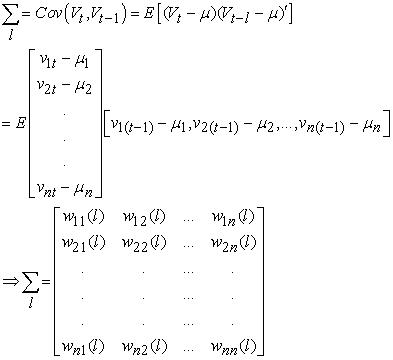(3)

where wij stands for the ijth matrix’s array of the cross-correlation function subjected to the lth time lag. Consequently, to construct the normalized CCFs (Rt) between a number of GPS stations for each year in three directions north-south, east-west and vertical, separately, we can define them as: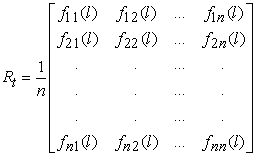(4)

where by considering equation (3), each component is defined in equation (4) as CCFs coefficient, thus: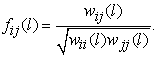(5)

Generally CCFs provides a statistical comparison of two sequences as a function of the timing lag between them 26, 41. Calculation of CCFs can yield us to display and then analyze the homogenous movements of the GPS stations caused by sensible earthquakes for a certain area, like central Alaska, every year.

2.3. Superimposed Decomposition of Wavelet Analysis

The wavelet analysis, especially the wavelet transform, is usually linked with a function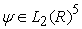while translation and dyadic dilation of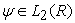constitute an orthonormal basis of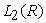10, 11, 13, 24, 25, 39, 44. The basis term for the discrete wavelet transform is: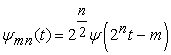(6)

where t denotes the time variable parameter, n and m belong to the Z set and the original wavelet function (ψ) should be subjected to the following compulsory integral as: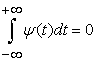(7)

In general, different forms of wavelet analysis have their own benefits and properties; such as harmonic wavelets which have a special spherical harmonics expansion and or triangulation, or based wavelets which would apply wavelets on a polyhedral approximation of an assumed sphere 12.

It is clear that the most important property of the wavelets is a time-frequency localization of their basis, similar to a windowed Fourier transform which allows time-frequency ability for representation of data.

Briefly, the result of the wavelet transform, as superimposed decomposition of a time series Rt, is an matrix of wavelet coefficients as Cmn where m is the time index and n is the scale (or layer) index. However, the wavelet coefficients in each layer can be considered as a time series with the sampling interval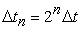, but it mostly varies due to the distribution’s type of original data. Moreover, by considering the detail of wavelet coefficients, the probability distribution function of the wavelet coefficients C(t) is a distribution that usually has zero mean value. Also, it is symmetric and very applicable to perform the superimposed decomposition in the wavelet domain 24, 39.

In the case of applying wavelet superimposed decomposition function into the time series of data which here are the annual cross-correlation of vertical GPS station’s displacements, the significant correlations can be estimated as a function of the wavelet layered index which would represent the different major frequency bands of that time series where is caused by recent earthquakes in central Alaska.

### 3. Data and Observations

Daily data time series for five continuous GPS stations, including displacements of the north-south, east-west and vertical components, with their corresponding standard deviations, have been prepared by the Plate Boundary Observatory (PBO) and International GNSS Service (IGS) for Alaska region. These continuous GPS stations are installed by UNAVCO and managed by PBO. Figure 1 shows the locations of the GPS stations in central Alaska.

For this study, we have considered 5 years of GPS data time series from January 1, 2008 to January 1, 2013 which these GPS stations are distributed over the central Alaska area in N-S direction. In particular, four of the 5 GPS stations (all stations except station SG27) are aligned in a perpendicular direction with respect to the North American Plate’s boundary. This may help to trace and investigate the response of the ground in the central part of Alaska; in Table 1 their names and locations are represented, geodetically.

On the other side, to compare GPS data time series with respect to the occurrences of earthquakes regimes, 5 years of recorded ground-based seismic observations for the whole Alaska (inside the area encompassing latitude 51°N to 71°N and longitude 140°W to 175°W) have been selected from the National Earthquake Information Center (NEIC) of the United State Geological Survey (USGS) (USGS-NEIC) data products. We sort the above data, which include the most recent earthquakes in Alaska region, with magnitude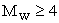and its corresponding depth from 0 to 150 km occurred during the period January 1, 2008 to January 1, 2013. According to USGS-NEIC seismometers, the total number of earthquakes which have taken place in Alaska region with a magnitude higher than Mw=2 during the whole 5 years is 11740. This number shows that the area is tectonically very active and the fault systems are subjected to a mutual and complex stress transfer pattern 8. Considering the stress-strain relation, the analysis of GPS data time series encompassing the seismic events would help to better understand how the stress in central Alaska has been accumulated, in the form of a “creep type” rock deformation which can be measured by GPS stations during inter-seismic periods, and then abruptly released during co-seismic phases.

### 4. Results and Discussions

4.1. GPS Stations Displacements, Correlations and Velocities

Time series displacements of geodetic GPS stations including AC15, AC20, ATW2, CLGO and SG27 station as well as the USGS’s earthquakes report are shown daily for 2008, 2009, 2010, 2011 and 2012 year in Figure 3, separately. The negative values illustrate the south, west and centripetal direction in north-south, east-west and vertical direction, respectively. It is clear that the number of earthquake’s events occurred with magnitude more than Mw=2 are more than Mw=4; as expected, there is a very good agreement between the earthquakes events and the horizontal and vertical response (in term of displacements) of the mentioned GPS stations. GPS stations displacement is in fact very sensitive to the both magnitude and depth of the earthquake, so that for instance, an earthquake with smaller magnitude but closer to the Earth’s surface can affect the GPS station’s movements in both horizontal and vertical directions largely rather than a higher magnitude but deeper earthquake. For example, for the day 231 of the year 2011, these five stations have horizontal displacements around 42 mm with depth of event on 81 km; but for the day 89 of the year 2012, they have 50 mm and 62 km in horizontal displacements and depth of event, respectively.

In order to take into account and analyze the vertical and the horizontal components of the GPS station displacement, the total displacement values of the GPS station have been computed for the years 2008, 2009, 2010, 2011 and 2012 (Figure 4). The total displacement value is derived based on the vectorial summation of the north-south, east-west and vertical directions. The displacements of these GPS stations originally follow the subduction movement induced by the Pacific Plate which is pushing away the continental North American Plate. The data calibration is here based on the North American reference frame (defined as Stable North American Reference Frame (SNARF) which more details about SNARF can be found in Blewitt et al. 6, 7) and thus the total velocity values have their maximum during 2012 year in compare to the other years.

To compute the average daily and annual velocity of GPS stations from 2008 to 2012, we apply a linear regression toward the mean values of the horizontal and vertical displacements. Table 2 and Table 3 show the average daily (mm/day) and annual (mm/year) velocity of AC15, AC20, ATW2, CLGO and SG27 GPS stations in horizontal and vertical directions, respectively. The negative values in the tables show the movements of GPS station along the south, west and or downward directions (or the combinations of them).

Similarly, the total GPS station velocity can be computed by using the horizontal and vertical velocities and applying the vectorial summation of these two components (Table 4). Considering all these GPS stations and their geographical location, distance length and orientation to the edge of Alaska’s fault, we found that AC15 GPS station has been affected by Pacific Plate more than the others based on having the maximum daily and yearly velocity from year 2008 to 2012, approximately.

Generally, the stress distribution, especially, after a large magnitude earthquake, is a very complicated and not completely solved problem since the characteristics of the Earth’s layers are spatially different. To take into account this, the correlation of GPS station displacements can distinguish the independent attitude of each station comparing to another one (Figure 5). The mean correlation values of the GPS stations would retrieve the shallowest displacements caused by the earthquake. Pointing out that as mentioned above, an earthquake with large magnitude but deeper, can affect less the surface layer with respect to a less strong but shallower one. The comparison of Figure 1 and Figure 5 provides a correspondence between the fluctuations of correlation values and the majority of recent earthquakes occurred in 2008, 2009, 2010, 2011 and 2012 year. In order to compute the correlation of GPS displacements, we considered each daily motion as a velocity vector with its own length and orientation in order to apply vectorial-based CCFs between the GPS station motions day by day from 2008 to 2012 year.

• Figure 3. Comparison of daily north-south, east-west and vertical direction of GPS stations displacements including AC15 (green), AC20 (black), ATW2 (red), CLGO (blue) and SG27 (magenta) and the USGS’s earthquakes report derived from seismometers across central Alaska for 2008, 2009, 2010, 2011 and 2012 year, respectively
• Figure 4. Total displacements for 2008 (red), 2009 (green), 2010 (blue), 2011 (black) and 2012 (magenta) year in AC15, AC20, ATW2, CLGO and SG27 GPS stations at central Alaska, respectively
• Figure 5. Mean Correlation of the GPS station displacements in horizontal and vertical direction for the stations AC15, AC20, ATW2, CLGO and SG27 in 2008, 2009, 2010, 2011 and 2012 year, respectively

In addition to Table 2 and Table 3, the Figure 6 and Figure 7 present the average annual velocities of GPS stations by a scaled velocity arrow for the years 2008, 2009, 2010, 2011 and 2012 in central Alaska, for horizontal and vertical components, respectively. In Figure 6, the velocities are oriented towards south-east. This is in accordance to the plate tectonic motion attitude 3, 5 since the North American plate uplifts the Pacific Plate 18.

4.2. Wavelet Analysis of GPS Stations Correlations

Figure 8 shows the results of applied superimposed decomposition wavelet in two numbers of coefficient level into normalized and then mean correlation of GPS vertical displacements for the years 2008, 2009, 2010, 2011 and 2012. The yellow color stands for the sudden changes in the vertical direction, while the red color represents the static case and fewer movements which are originally sensed by GPS station. In fact, the red and blue colored graphs show the first and second number of coefficient’s orders in the superimposed decomposition wavelet for normalized vertical displacement of the GPS stations signals. The light-green and dark-green graphs present these two levels only for superimposed decomposition of the vertical displacements.

In addition to this, Figure 8 demonstrates the ability of wavelet approach to detect the signature of the sudden fluctuations occurred over the Earth crustal surface, in particular, the vertical displacements given by a succession of features, as yellow colored columns on a precise sensing module applied in two-level scales. Although the wavelet proposed at level 2 is more sensitive than level 1, but a small irregularity in the signal (here as mean correlation of the vertical displacements for GPS stations in central Alaska yearly from 2008 to 2012) would affect it largely by considering the similar situations of the wavelet functions in both two levels 30. In fact, the designed wavelet superimposed decomposition at level 1 is more stable when facing sudden movements and may neglect very high abnormality in a short transit time.

• Figure 6. Average annual horizontal velocity vectors of GPS stations including AC15, AC20, ATW2, CLGO and SG27 at central Alaska during 2008, 2009, 2010, 2011 and 2012 year in the Stable North American Reference Frame (SNARF version.1.0)

As demonstrated in equation (6) and (7), the probability distribution function of the wavelet coefficients has zero mean value with symmetrical normalized histograms. Therefore, Figure 9 presents the histograms of superimposed decomposition of three signals shown in Figure 8 including the main function, wavelet analysis in level 1 and also in level 2 for 2008, 2009, 2010, 2011 and 2012 year, respectively.

• Figure 7. Average annual vertical velocity of GPS stations including AC15, AC20, ATW2, CLGO and SG27 at central Alaska during 2008, 2009, 2010, 2011 and 2012 year in the Stable North American Reference Frame (SNARF version.1.0)
• Figure 8. Normalized and superimposed decomposition of mean correlations of the GPS station vertical displacements based on wavelet approach including AC15, AC20, ATW2, CLGO and SG27 GPS stations during 2008, 2009, 2010, 2011 and 2012 year, respectively
• Figure 9. Normal distribution histogram of superimposed decomposition and coefficient levels 1 and 2 for vertical displacement of the GPS stations based on wavelet approach during 2008, 2009, 2010, 2011 and 2012 year, respectively

It is clear that the standard deviation for superimposed decomposition wavelet analysis in level 2 is bigger than level 1. This also can be understood from Figure 8 where the color bars in level 2 are more dispersed than level 1. Similarly, the bias for level 1 is less than level 2. The reason is that level 1 has less sensitivity to the noise and thus level 2 would stray more from the zero mean value rather than level 1.

### 5. Summary and Conclusions

In this study, we discussed the effects of recent Earth’s surface movements in central Alaska region measured by five selected GPS stations, through CCFs values and an invented superimposed decomposition of wavelet analysis in level number 1 and number 2. As mentioned in the results and discussions section, generally the analysis of Earth crustal movements is a very complicated issue. In order to better understand the crustal displacements, it is suggested here that we can consider more sensitive approaches and techniques such as CCFs and superimposed decomposition of wavelet analysis (e.g. Figure 5, Figure 8 and Figure 9). Furthermore, in this study, the daily and annual velocities of the mentioned GPS stations in central Alaska region are computed and analyzed precisely in the Stable North American Reference Frame (SNARF) (Table 2, Table 3 and Table 4). Referring to superimposed decomposition wavelet analysis and its histograms, for the years 2009 and 2010, we found a very good convergence in the solution of the corresponding designed wavelet for those years and the earthquake regimes. This can also be inferred from the CCFs results (e.g. Figure 5) and normal histograms of each component and levels (Figure 9). Moreover, comparison of Figure 2, Figure 4 and Figure 5 represents that those earthquakes which could significantly affect on GPS stations movements vertically and horizontally, they can be retrieved by the designed wavelet superimposed decomposition. It should be noted here that the wavelet function is more sensitive to horizontal displacements rather than vertical ones due to existence of two dimensions (north-south and east-west directions) compare to the single dimension for vertical direction.

As mentioned, the wavelet functional analysis can localize information in both time and frequency space, simultaneously; and thus it is a very useful tool with low amounts of computation for seismic data analysis rather than existing GPS displacement analysis lonely. Moreover, the designed superimposed decomposition wavelets have very good capabilities in order to retrieve the seismic waves reached to the Earth’s surface for all the selected GPS stations in these 5 years GPS station’s displacement daily observations. However, the Earth’s surface vibration with the possible responses from its interior layers together are a very complex issue to be examined through non-parametric solutions; then further studies and researches are necessary, in particular, for central Alaska region where the seismic movements are accommodated by several faults where the presence of volcanic activities would make this territory even more complicated one, especially, from the kinematical aspect.

Additionally, since the analysis of Earth crust movements is really localized and the selection of that suitable wavelet decomposition has an essential role for such studies, here we discussed a useful wavelet analysis based on superimposed decomposition in two levels. The presented results encourage further studies in order to apply more precise and applicable tools to sense and compute the Earth surface’s crustal movements (even by considering separately the pre-seismic, co-seismic and post-seismic deformations) which would result in fluctuations in the GPS station time series displacement.

### Acknowledgements

The authors are grateful to the GPS stations data which are based on data, equipment and engineering services provided by the Plate Boundary Observatory operated by UNAVCO for EarthScope (http://www.earthscope.org) and supported by the National Science Foundation (No. EAR-0350028 and EAR-0732947).

### ReferencesThis work is licensed under a Creative Commons Attribution 4.0 International License. To view a copy of this license, visit http://creativecommons.org/licenses/by/4.0/